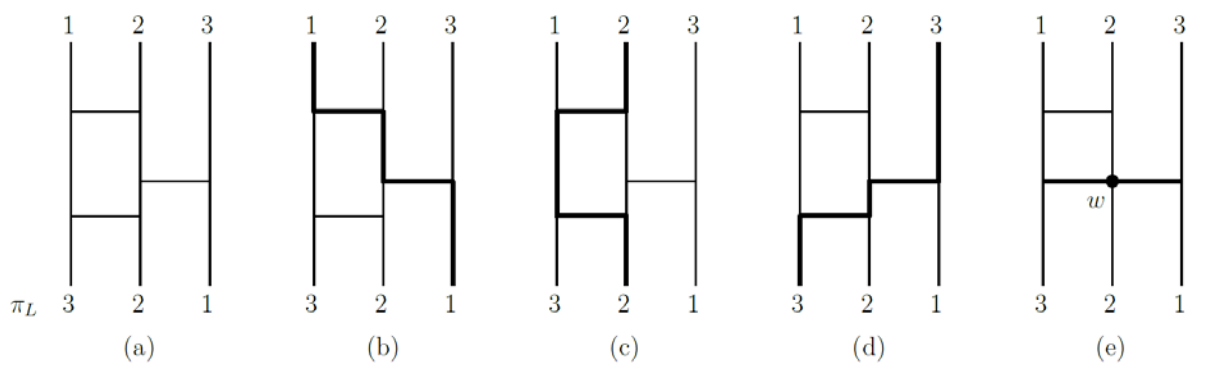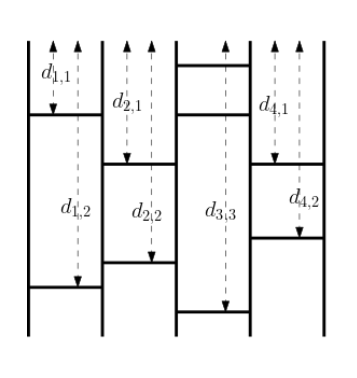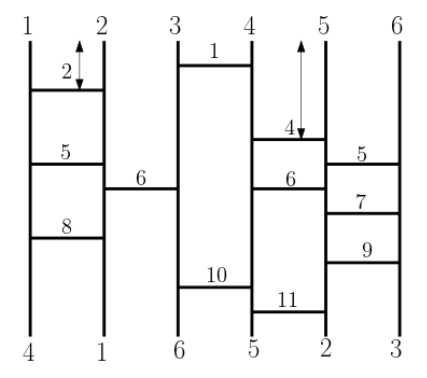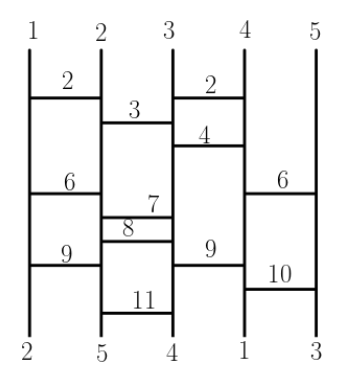시간 제한메모리 제한제출정답맞힌 사람정답 비율
1 초 512 MB193967651.701%

## 문제

Consider a situation that we need to distribute n gifts to n people fairly and randomly. For this purpose one ancient technique is popular in Asia, and is usually used to represent a random permutation. Chinese call it Ghost Leg (畫鬼腳), Japanese Budda Lots (あみだくじ) and Korean Ladder Game (사다리타기). We first start with some terms. This ladder consists of several vertical poles and horizontal bars connecting two adjacent vertical poles. From the top of each vertical pole, a path is traced through the ladder using the following three steps:

1. When tracing a vertical pole, continue downwards until an end of the first bar is reached, then continue along the bar.
2. When tracing a bar, continue along it until the end of the bar is reached, then continue down the vertical pole.
3. Repeat Step 1 and Step 2 until the bottom of a vertical pole is reached.

Figure D.1(a) shows a ladder L with three vertical poles and three bars. The vertical poles are numbered with 1, … , n from left to right. The paths of tracing these three vertical poles are shown in (b), (c), and (d). The input (1, 2, 3) is permuted finally to πL = (3, 2, 1) by the ladder L. Note that we do not allow the case that two immediately adjacent horizontal bars meet at some point (like w in Figure D.1(e)) because there is no unique way in tracing at the point.You are given a ladder L which achieves a permutation πL. If the permutation πL is unchanged after removing a set of bars containing a particular bar, then the bar is said to be redundant for πL. Your task is to find all redundant bars for πL. This is equivalent to constructing a minimal ladder by removing all redundant bars from L. In the minimal ladder of L, the removal of any non-empty set of bars from the ladder gives a different permutation from πL.

## 입력

Your program is to read from standard input. The input starts with a line containing one integers n (3 ≤ n ≤ 50), where n is the number of vertical poles. The vertical poles are ordered from left to right. Let di,j denote the depth of the j-th bar between the i-th and (i + 1)-th poles from the top, which is an integer between 1 and 1,000. In the following n − 1 lines, the i-th line contains the sequence of depths di,j, where 1 ≤ i < n, j ≥ 1 and di,j < di,j+1. Notice that this depth sequence is ended with zero (0), which is not a depth but just a marker to indicate the end of the sequence. See Figure D.2 for illustration.(a) Depth di,j of the bars (b) Sample input 1 (c) Sample input 2

Figure D.2: Depths of the bars between two adjacent poles in the ladder.

## 출력

Your program is to write to standard output. Print a set of the bars to be remained in a minimal ladder for πL. The first line should contain k, the number of the bars remained in the minimal ladder. Then, each line of the following k lines should contain two indices i and j of di,j of the bar in the minimal ladder. Note that the minimal ladder is not unique.

## 예제 입력 1

6
2 5 8 0
6 0
1 10 0
4 6 11 0
5 7 9 0


## 예제 출력 1

8
3 1
4 1
5 1
2 1
4 2
1 3
3 2
4 3


## 예제 입력 2

5
2 6 9 0
3 7 8 11 0
2 4 9 0
6 10 0


## 예제 출력 2

6
1 1
3 1
2 1
4 1
3 3
2 4


## 출처

• 스페셜 저지를 만든 사람: alex9801
• 잘못된 조건을 찾은 사람: jh05013